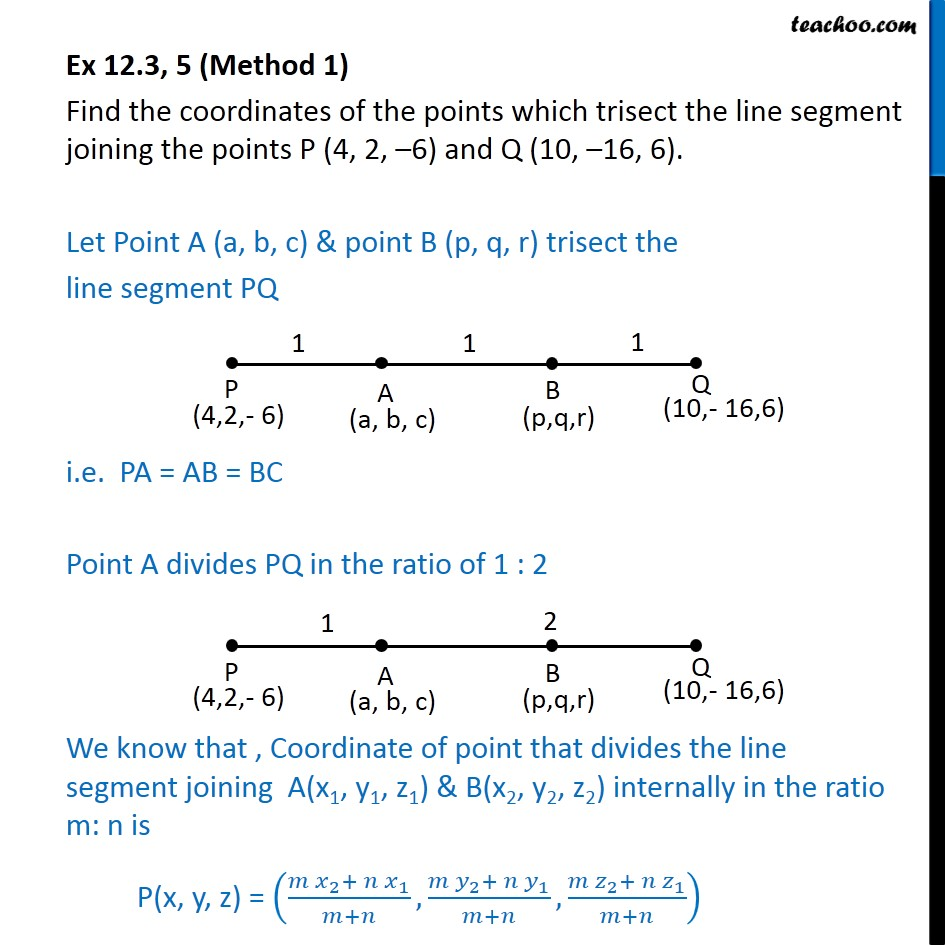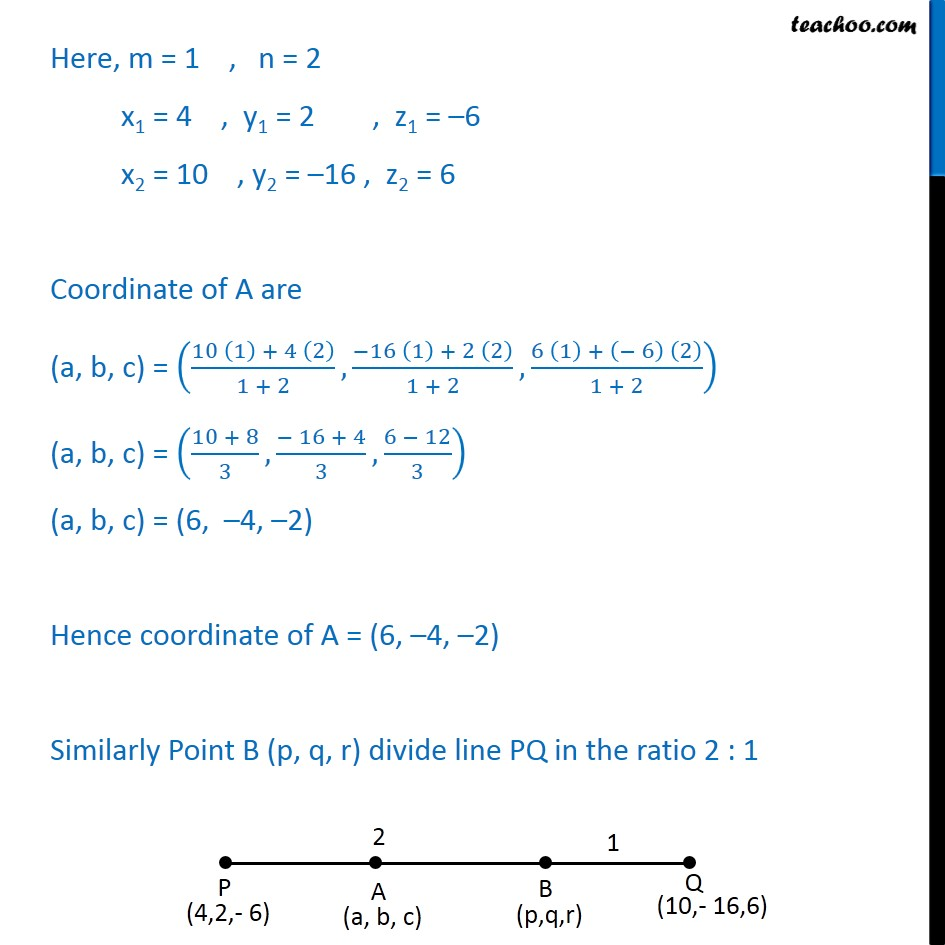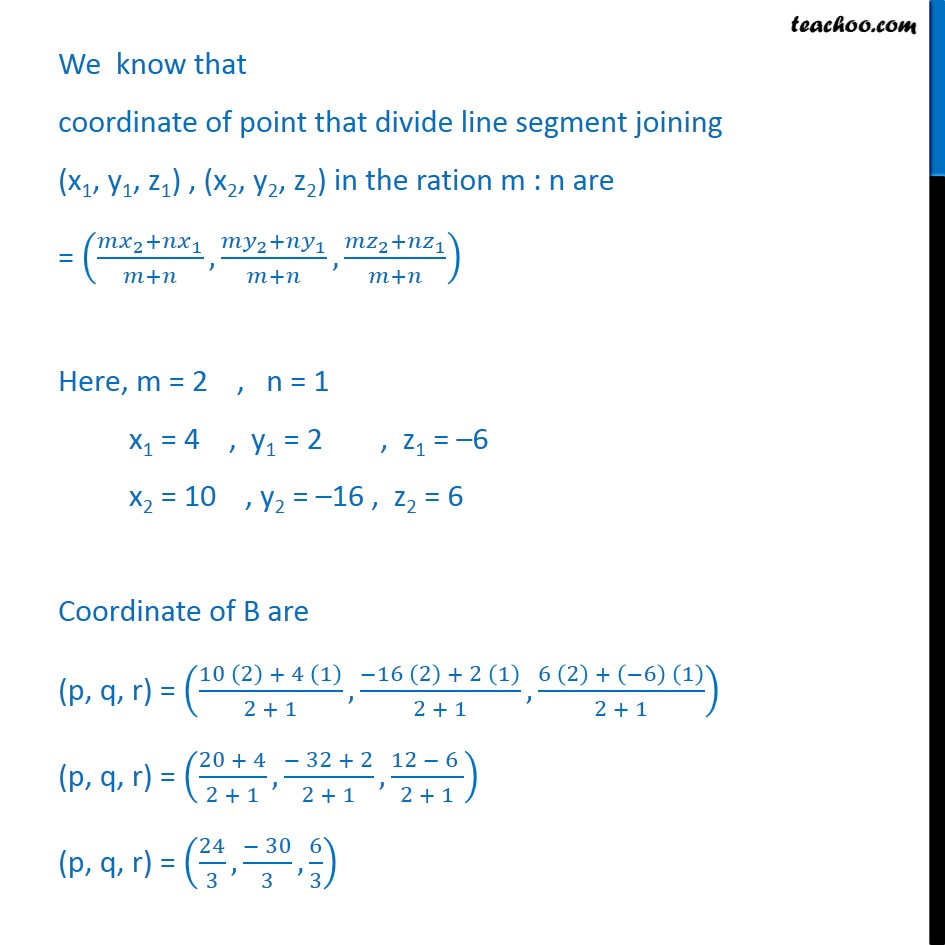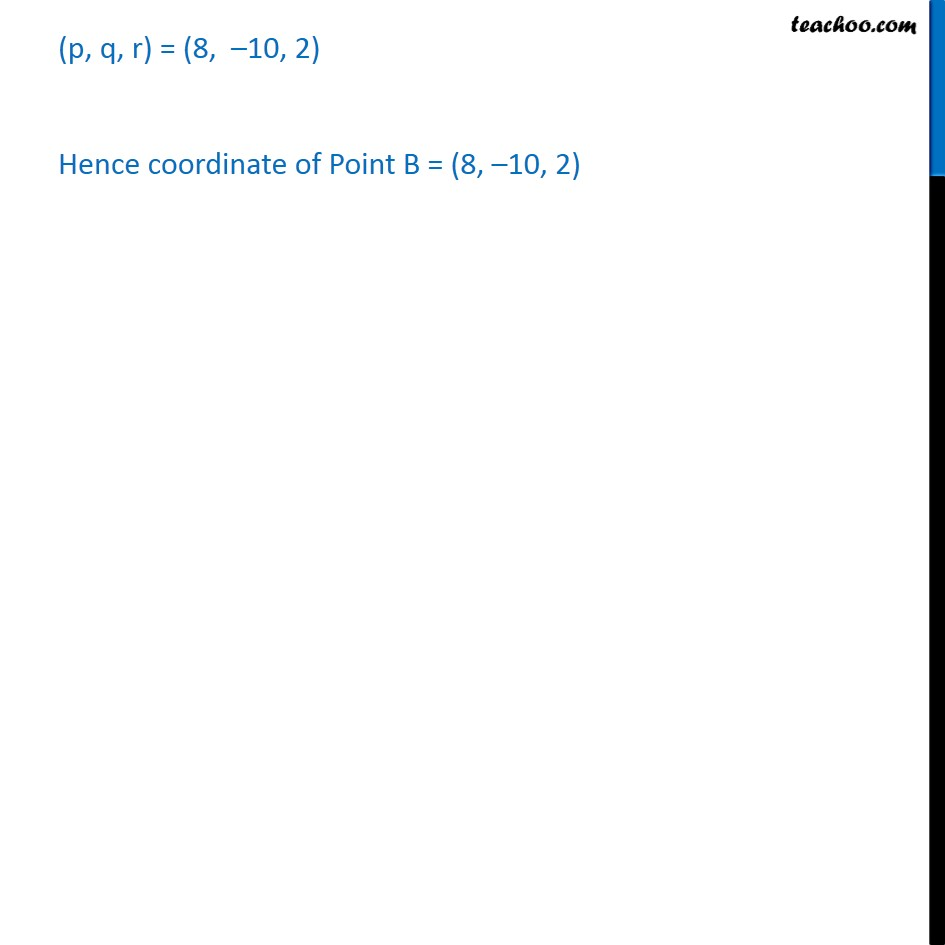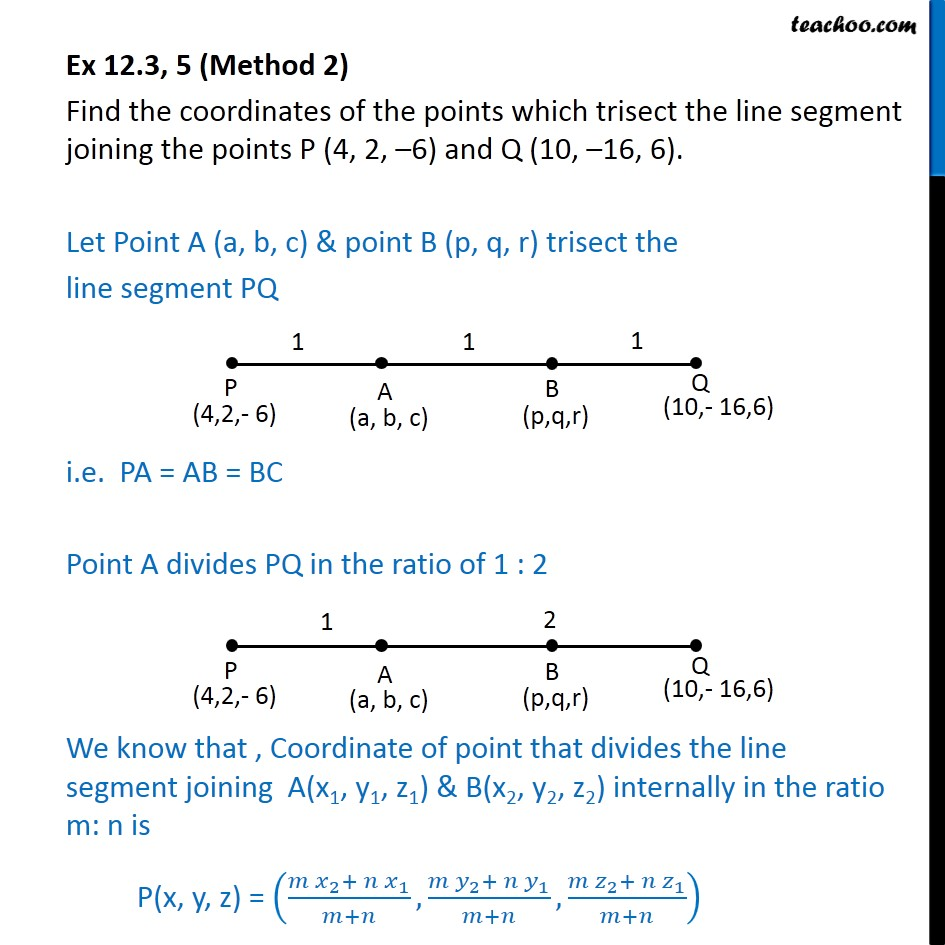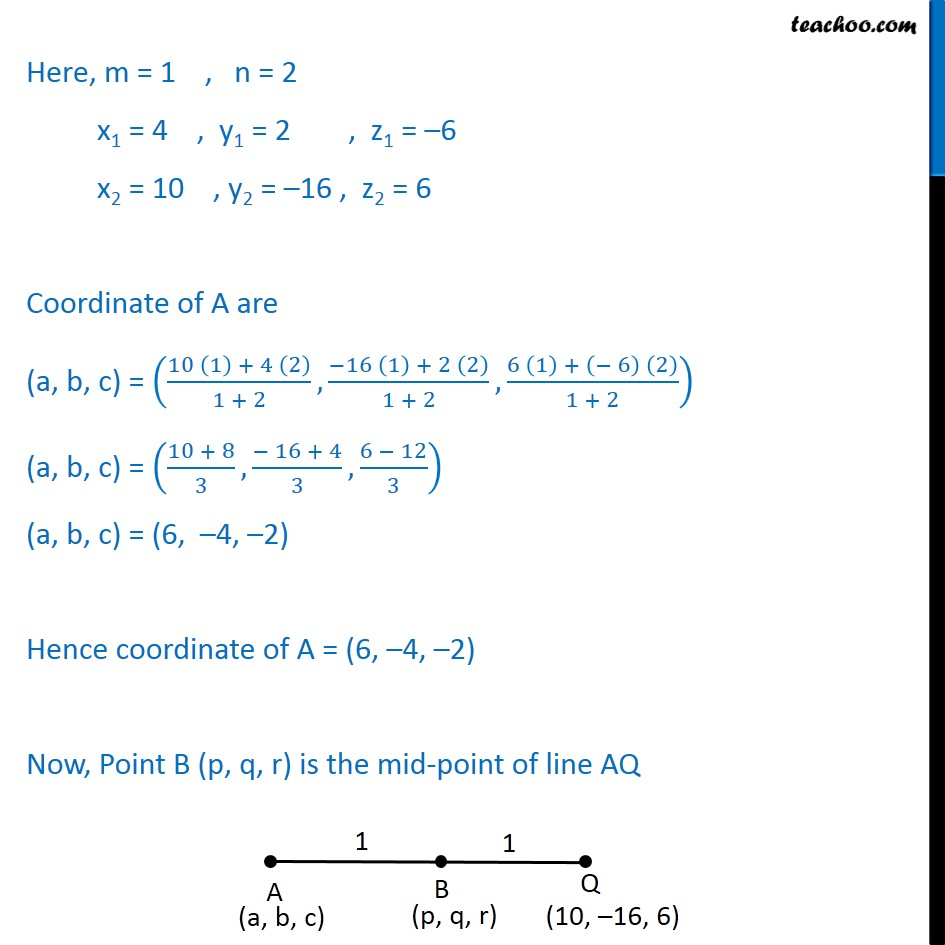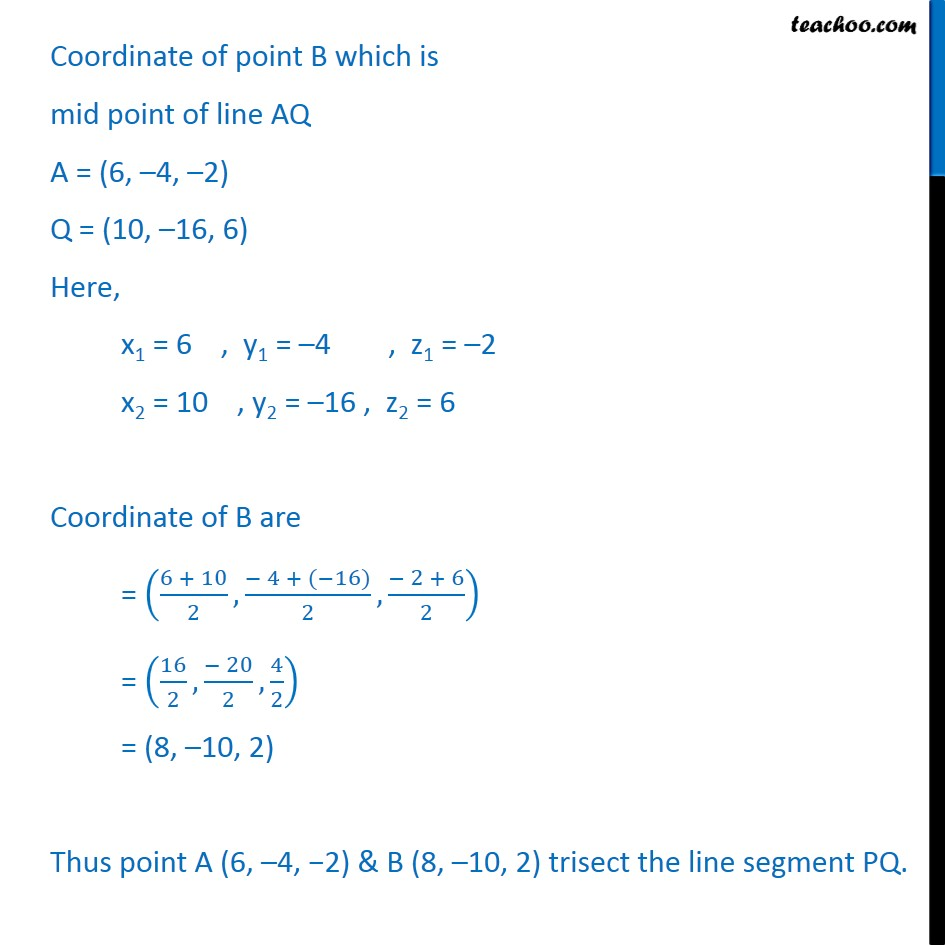1. Chapter 12 Class 11 Introduction to Three Dimensional Geometry
2. Serial order wise
3. Ex 12.3

Transcript

Ex 12.3, 5 (Method 1) Find the coordinates of the points which trisect the line segment joining the points P (4, 2, –6) and Q (10, –16, 6). Let Point A (a, b, c) & point B (p, q, r) trisect the line segment PQ i.e. PA = AB = BC Point A divides PQ in the ratio of 1 : 2 We know that , Coordinate of point that divides the line segment joining A(x1, y1, z1) & B(x2, y2, z2) internally in the ratio m: n is P(x, y, z) = ((〖𝑚 𝑥〗_2+〖 𝑛 𝑥〗_1)/(𝑚+𝑛),(〖𝑚 𝑦〗_2+〖 𝑛 𝑦〗_1)/(𝑚+𝑛),(〖𝑚 𝑧〗_2+〖 𝑛 𝑧〗_1)/(𝑚+𝑛)) Here, m = 1 , n = 2 x1 = 4 , y1 = 2 , z1 = –6 x2 = 10 , y2 = –16 , z2 = 6 Coordinate of A are (a, b, c) = ((10 (1) + 4 (2))/(1 + 2),(−16 (1) + 2 (2))/(1 + 2),(6 (1) + (− 6) (2))/(1 + 2)) (a, b, c) = ((10 + 8)/3,(− 16 + 4)/3,(6 − 12)/3) (a, b, c) = (6, –4, –2) Hence coordinate of A = (6, –4, –2) Similarly Point B (p, q, r) divide line PQ in the ratio 2 : 1 We know that coordinate of point that divide line segment joining (x1, y1, z1) , (x2, y2, z2) in the ration m : n are = ((〖𝑚𝑥〗_2+〖𝑛𝑥〗_1)/(𝑚+𝑛),(〖𝑚𝑦〗_2+〖𝑛𝑦〗_1)/(𝑚+𝑛),(〖𝑚𝑧〗_2+〖𝑛𝑧〗_1)/(𝑚+𝑛)) Here, m = 2 , n = 1 x1 = 4 , y1 = 2 , z1 = –6 x2 = 10 , y2 = –16 , z2 = 6 Coordinate of B are (p, q, r) = ((10 (2) + 4 (1))/(2 + 1),(−16 (2) + 2 (1))/(2 + 1),(6 (2) + (−6) (1))/(2 + 1)) (p, q, r) = ((20 + 4)/(2 + 1),(− 32 + 2)/(2 + 1),(12 − 6 )/(2 + 1)) (p, q, r) = (24/3,(− 30)/3,6/3) (p, q, r) = (8, –10, 2) Hence coordinate of Point B = (8, –10, 2) Ex 12.3, 5 (Method 2) Find the coordinates of the points which trisect the line segment joining the points P (4, 2, –6) and Q (10, –16, 6). Let Point A (a, b, c) & point B (p, q, r) trisect the line segment PQ i.e. PA = AB = BC Point A divides PQ in the ratio of 1 : 2 We know that , Coordinate of point that divides the line segment joining A(x1, y1, z1) & B(x2, y2, z2) internally in the ratio m: n is P(x, y, z) = ((〖𝑚 𝑥〗_2+〖 𝑛 𝑥〗_1)/(𝑚+𝑛),(〖𝑚 𝑦〗_2+〖 𝑛 𝑦〗_1)/(𝑚+𝑛),(〖𝑚 𝑧〗_2+〖 𝑛 𝑧〗_1)/(𝑚+𝑛)) Here, m = 1 , n = 2 x1 = 4 , y1 = 2 , z1 = –6 x2 = 10 , y2 = –16 , z2 = 6 Coordinate of A are (a, b, c) = ((10 (1) + 4 (2))/(1 + 2),(−16 (1) + 2 (2))/(1 + 2),(6 (1) + (− 6) (2))/(1 + 2)) (a, b, c) = ((10 + 8)/3,(− 16 + 4)/3,(6 − 12)/3) (a, b, c) = (6, –4, –2) Hence coordinate of A = (6, –4, –2) Now, Point B (p, q, r) is the mid-point of line AQ Coordinate of point B which is mid point of line AQ A = (6, –4, –2) Q = (10, –16, 6) Here, x1 = 6 , y1 = –4 , z1 = –2 x2 = 10 , y2 = –16 , z2 = 6 Coordinate of B are = ((6 + 10)/2,(− 4 + (−16))/2,(− 2 + 6)/2) = (16/2,(− 20)/2,4/2) = (8, –10, 2) Thus point A (6, –4, −2) & B (8, –10, 2) trisect the line segment PQ.

Ex 12.3